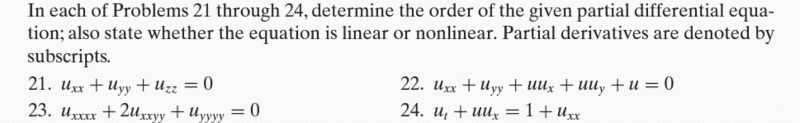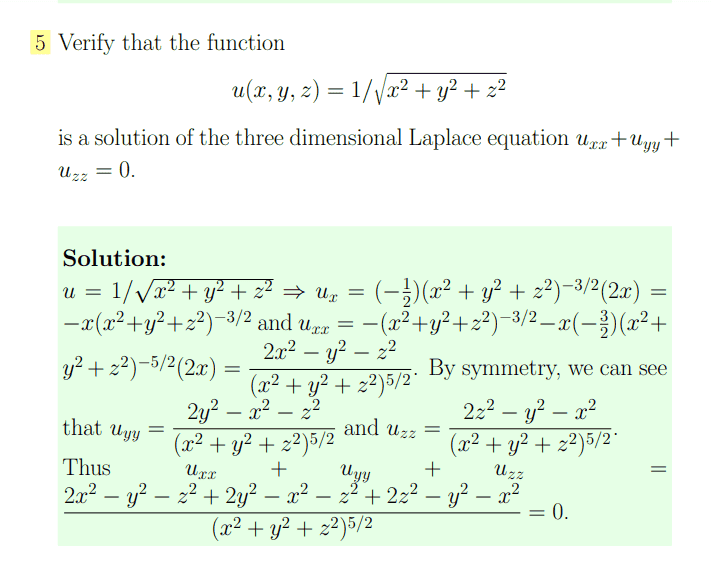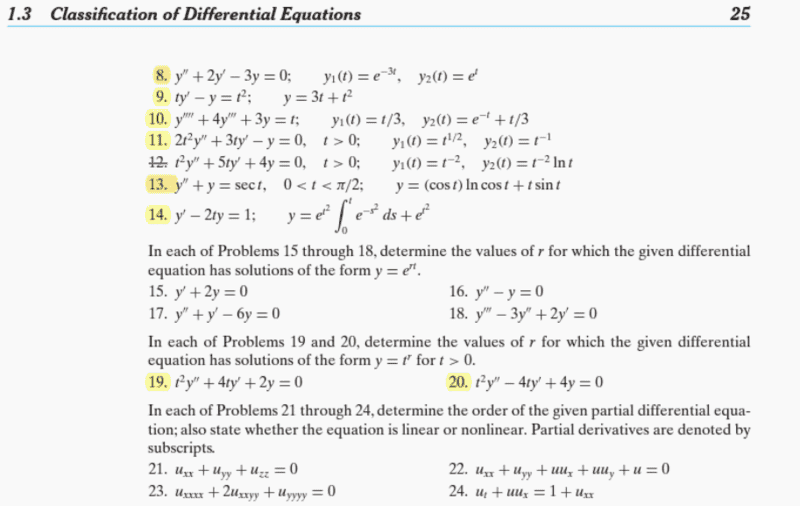# Determine the order of differentiation for this partial differential eqn

• karush
In summary, the conversation discusses the equivalence between two notations for partial derivatives and the concept of linear and non-linear differential equations. The participants also mention using observation to identify linear equations and provide examples. f

#### karush

Gold Member
MHB
Homework Statement
determine the order of the given partial differential equation;also state whether the equation is linear or nonlinear
Relevant Equations
The ordinary differential equation is said to be linear if F is a linear function of the variables y,y,...,y(n); a similar definition applies to partial differential equations.
The order of a differential equation is the order of the highest derivative that appears in the equation.ok I posted this a few years ago but replies said there was multiplication in it so I think its a mater of format
##\dfrac{\partial u^2}{\partial x\partial y}## is equivalent to ##u_{xy}##

textbook

Last edited:
here is an example I found from online ... but its probably beyond what my OP is asking forFor "Relevant Equations", you should not just put "definitions". You should put your exact definition of the order of a PDE.

Also use double pound ## for inline math

Also use double pound ## for inline math
double pound is not very collaborate with other latex editors this is the only forum I know of that asks that

ok it looks these are just observation problems but ? about linear non linear pare

double pound is not very collaborate with other latex editors this is the only forum I know of that asks that
But you're here, and that's the way it works here...

"When in Rome, ..."

•WWGD
##\dfrac{\partial u^2}{\partial x\partial y}## is equivalent to ##u_{xy}##
If memory serves, the two notations are not the same; i.e., the order in which partials are taken is different.
The above should read "##\dfrac{\partial u^2}{\partial x\partial y}## is equivalent to ##u_{yx}##", not ##u_{xy}## as you wrote.
In the first notation, you first take the partial with respect to y, and then take the partial of that with respect to x. In the second notation, you take the partial with respect to the first variable listed, and then with the second.

Last edited:
•karush and berkeman
It's not clear to me what we are being asked to do. The first two posts are not about the same issue regarding PDEs. And there is no attempt to answer either.

•Orodruin, SammyS and berkeman
If memory serves, the two notations are not the same; i.e., the order in which partials are taken is different.
The above should read "##\dfrac{\partial u^2}{\partial x\partial y}## is equivalent to ##u_{yx}##", not ##u_{xy}## as you wrote.
In the first notation, you first take the partial with respect to y, and then take the partial of that with respect to x. In the second notation, you take the partial with respect to the first variable listed, and then with the second.
IIRC, the two are barely-ever different from each other.

•pbuk
It's not clear to me what we are being asked to do. The first two posts are not about the same issue regarding PDEs. And there is no attempt to answer either.
it basically to solve just by observation like 2 order and linear no calculation is needed

it basically to solve just by observation like 2 order and linear no calculation is needed
In your first post, what do you think the answers are? We can comment on that.

IIRC, the two are barely-ever different from each other.
By Schwarz's theorem they are the same, providing both partial derivatives are continuous differentiable[/edit].

•WWGD
In your first post, what do you think the answers are? We can comment on that.
ok I would like that I don't know how to discern linear for non Linear from observation
the yellow highlighted problems are ones I have done but want to do the 15-24

the link to the whole Boyce textbook in the OP if need.

BTW I am retired so just doing this on my own might audit de at UHM next year•